Truncated Tetrahedron (adapted from Leonardo da Vinci)

This video is an adaptation of the drawing that Leonardo da Vinci made of the truncated tetrahedron (exacedron abscisus vacuus) for Luca Pacioli's book 'De Divina Proportione'.

Pacioli wrote about the truncated tetrahedron (Spanish translation):

"El tetraedro despuntado o absciso sólido plano o hueco está contenido por dieciocho líneas que forman treinta y seis ángulos superficiales y doce sólidos; por tanto, lo circundan ocho bases, cuatro de las cuales son hexagonales, es decir, de seis lados iguales, y cuatro triangulares, es decir, de tres lados, igualmente equiláteras y equiángulas. Pero, de estas dieciocho líneas, doce son comunes a las bases triagulares y hexagonales; y éstas son todas propias de los hexágonos, porque necesariamente los cuatro hexágonos juntos forman con algunos de sus lados los cuatro triángulos, como la experiencia de su propia forma material nos muestra de modo evidente. Y deriva del precedente -el tetraedro- mediante el corte uniforme de sus lados en tres partes iguales."
('La divina proporción' de Luca Pacioli, page 90, Spanish translation by Juan Calatrava, Editorial Akal, 4th edition, 2008)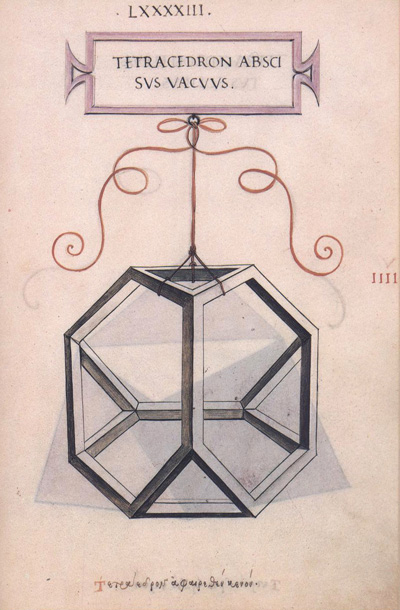Leonardo da Vinci's drawing of the truncated tetrahedron (tetracedron abscisus vacuus) for Luca Pacioli's book 'De divina proportione'. (There is an Spanish version, 'La divina proporción' Editorial Akal. Image used with permission of Editorial Akal).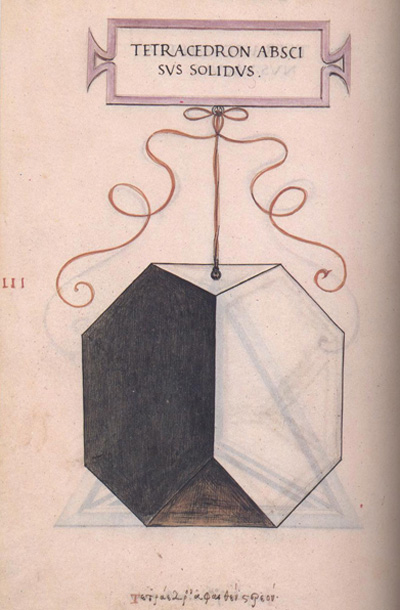Leonardo da Vinci's drawing of the truncated tetrahedron (tetracedron abscisus solidus) for Luca Pacioli's book 'De divina proportione'. (There is an Spanish version, 'La divina proporción' Editorial Akal. Image used with permission of Editorial Akal).

If you play with the interactive application you can get images like these: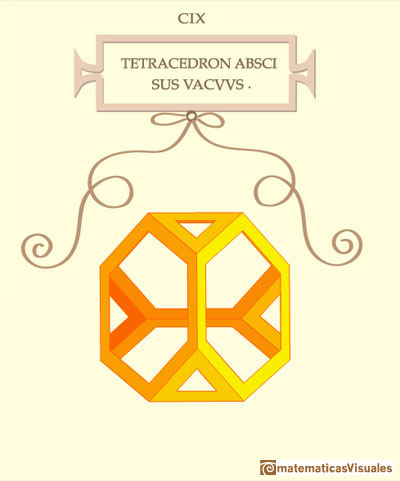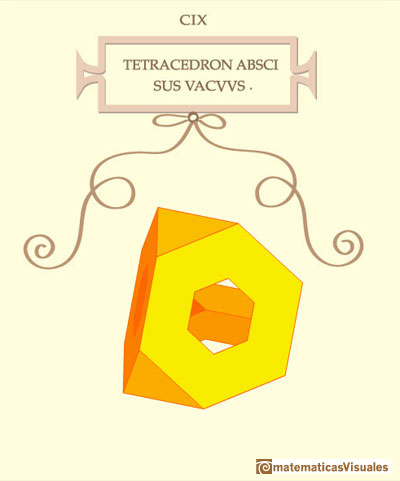REFERENCES

Luca Pacioli - La divina proporción - Ediciones Akal, 4th edition, 2004. Spanish edition of 'De divina proportione'. Translation by Juan Calatrava.
Leonardo da Vinci's Geometric Sketches Frank J. Swetz's article in MathDl, Loci:Convergence.
Leonardo da Vinci's Polyhedra George Hart's excellent website about polyhedra.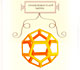Leonardo da Vinci made several drawings of polyhedra for Luca Pacioli's book 'De divina proportione'. Here we can see an adaptation of the dodecahedron.Leonardo da Vinci made several drawings of polyhedra for Luca Pacioli's book 'De divina proportione'. Here we can see an adaptation of the truncated octahedron.Leonardo da Vinci made several drawings of polyhedra for Luca Pacioli's book 'De divina proportione'. Here we can see an adaptation of the cuboctahedron.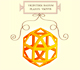Leonardo da Vinci made several drawings of polyhedra for Luca Pacioli's book 'De divina proportione'. Here we can see an adaptation of the rhombicuboctahedron.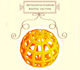Leonardo da Vinci made several drawings of polyhedra for Luca Pacioli's book 'De divina proportione'. Here we can see an adaptation of the Campanus' sphere.The truncated tetrahedron is an Archimedean solid made by 4 triangles and 4 hexagons.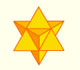The stellated octahedron was drawn by Leonardo for Luca Pacioli's book 'De Divina Proportione'. A hundred years later, Kepler named it stella octangula.The compound polyhedron of a cube and an octahedron is an stellated cuboctahedron.It is the same to say that the cuboctahedron is the solid common to the cube and the octahedron in this polyhedron.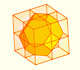Using eight half cubes we can make a truncated octahedron. The cube tesselate the space an so do the truncated octahedron. We can calculate the volume of a truncated octahedron.One eighth of a regular dodecahedon of edge 2 has the same volume as a dodecahedron of edge 1.The volume of a tetrahedron is one third of the prism that contains it.The first drawing of a plane net of a regular tetrahedron was published by Dürer in his book 'Underweysung der Messung' ('Four Books of Measurement'), published in 1525 .The volume of an octahedron is four times the volume of a tetrahedron. It is easy to calculate and then we can get the volume of a tetrahedron.The truncated octahedron is an Archimedean solid. It has 8 regular hexagonal faces and 6 square faces. Its volume can be calculated knowing the volume of an octahedron.A cuboctahedron is an Archimedean solid. It can be seen as made by cutting off the corners of a cube.A cuboctahedron is an Archimedean solid. It can be seen as made by cutting off the corners of an octahedron.These polyhedra pack together to fill space, forming a 3 dimensional space tessellation or tilling.When you truncate a cube you get a truncated cube and a cuboctahedron. If you truncate an octahedron you get a truncated octahedron and a cuboctahedron.You can chamfer a cube and then you get a polyhedron similar (but not equal) to a truncated octahedron. You can get also a rhombic dodecahedron.